# Regression Objective and Evaluation Functions

Objective function vs Evaluation function

The objective function is the target that your model tries to optimize when training on a dataset.

The Evaluation function (or evaluation metrics) is, as suggested by its name, a function to evaluate the performance of a Machine learning model on a dataset.

New researchers often get confused about objective functions and evaluation functions. Their difference is: the objective function is perceived by your model/algorithm, it targets to optimize the objective function. On the other hand, the evaluation function is only observed by the researchers themselves and is evaluated after the training complete.

A common question is: Why do we care about the evaluation function but have the model to optimize the objective function? If we want the evaluation function, why don’t we use it as the target for our model to optimize?

The answer is: yes, in the perfect world, a separate objective function should not exist, our model should optimize the evaluation function, which is the function we – researchers really care about. But in our world, it is not so easy. Some evaluation function is not optimizable by the machine, which is why we need an objective function to act as a proxy function to approximate the evaluation function. To go a bit farther, the reason for being not optimizable is because they are not differentiable, which is a needed condition for optimization algorithms like Gradient Descent.

On a side note, a model can have only 1 objective function but can have many evaluation functions. It is also advisable for researchers to evaluate model performance on various points of view (various evaluation functions).

Every objective function can work as an evaluation function, but not vice versa.

There are other terms that are closely related to Objective function, like Loss function or Cost function.

In high-level usage, you can just assume that those terms have the same meaning and are just other names for Objective function.

But in some literature, the authors may use them a little bit differently:

• The Loss function is sometimes referred to as a function to compute the error of your prediction on 1 data point, while
• The Cost function measures the total error of your predictions on the entire dataset.

The functions

Below, I will list out some of the most common objective/evaluation functions for regression models. Note that these functions measure the error of the whole dataset, not just an individual sample like loss functions.

Depending on the problem we want to solve that we choose a suitable objective function (and one or more evaluation functions).

Mean Absolute Error

Mean Absolute Error (MAE) is also called the L1 cost function.

MAE =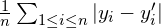,

where:
n is the number of samples,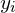is the true response of the i-th sample,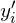is the predicted response of the i-th sample.

Value of MAE is in range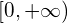.

For our model to be better, we should minimize MAE.

MAE can be used as an Objective function.

Mean Squared Error

Mean Squared Error (MSE) is also called the L2 cost function.

MSE =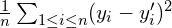,

where:
n is the number of samples,is the true response of the i-th sample,is the predicted response of the i-th sample.

Value of MSE is in range.

For our model to be better, we should minimize MSE.

MSE can be used as an Objective function.

Max Absolute Error

Max Absolute Error (MaxAE) is also called the L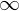cost function.

MaxAE =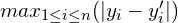,

where:
n is the number of samples,is the true response of the i-th sample,is the predicted response of the i-th sample.

Value of MaxAE is in range.

For our model to be better, we should minimize the MaxAE.

MaxAE cannot be used as an Objective function.

Root Mean Squared Error

RMSE =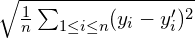,

where:
n is the number of samples,is the true response of the i-th sample,is the predicted response of the i-th sample.

Value of RMSE is in range.

For our model to be better, we should minimize RMSE.

RMSE can be used as an Objective function.

R-Squared

R-squared (or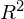or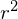) represents how much variance of the response variable is predictable from the predictor variables.

Suppose:is the mean of all observed responses, we have: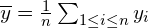The total sum of squares, which measure the variance of responses: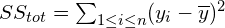The sum of squares of residual (residual is the difference between true response and predicted response, sometimes this term can be used interchangeably with error):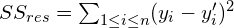The R-squared is computed by: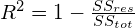.

Normally, value ofis in range [0, 1].can only be negative if the model you use is worse than a simple model that gives the output as the mean of the responses for any sample.

A value of, for e.g. 0.78, means that using our model, 78% of the difference in the response variable can be explained by the predictor variables.

For our model to be better, we should maximize.

Note thatshould not be used for non-linear models since those models are extremely complex and can perfectly-fit almost any input data, usingwill over-rate those models’ strength. Even for linear models,is not enough to determine if a model is acceptable or not.cannot be used as an Objective function.

A disadvantage of R-squared is that it prefers models with a higher number of predictor variables. Notice the more the predictors, the more your linear model will over-fit the training data, which results in a higher. In other words, when we want a measurement that gives higher value if the model is better (not just on the training data, but in general), R-squared gives higher value when the model fits the training data better, even if it over-fits.

What should be emphasized here is thatwill only be higher or equal when we add more predictors to the model, which is not good because we cannot compare 2 models with a different number of predictors. Hence, the Adjustedwas born to solve this problem.

For Adjusted, adding a new predictor will only increase Adjustedif that predictor increases the model’s performance more than expected by chance. That is to say, adding a good predictor will help the Adjustedincrease, while adding a bad one will make Adjusteddecrease.

Adjusted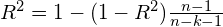,

where:
n is the number of samples,
k is the number of predictor variables in the model.

You may also noticed that:

Adjusted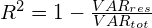,

where: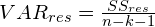is the unbiased variance of residuals.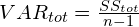is the unbiased variance of responses.

Adjustedis usually in range [0, 1] and sometimes can be negative. Adjusted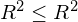.

For our model to be better, we should maximize the Adjusted.

Adjustedcannot be used as an Objective function.

Predicted R-Squared

Predictedis simplyof the testing data. While usingor Adjusted, we don’t separate our data to a training set and a testing set, yet, with Predicted, we do.

We can use many methods to split data into training and testing sets (e.g. random split, k-fold cross-validation). K-fold is preferred because it is stronger over over-fitting. Here is a guideline for splitting.

The idea here is: we training our model on the training set, and Predictedis themeasured on the testing set. By calculatingon a separated set of data, we can be more confident with the generalization power of the model.

Note that how we split the data is very important. If any information on the testing data is leaked to the training data, it will ruin our measurement.

Predictedcannot be used as an Objective function.

Mean Squared Log Error

MSLE =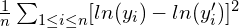,

where:
n is the number of samples,is the true response of the i-th sample,is the predicted response of the i-th sample.

MSLE is often used when the response can be exponentially large. E.g. when your problem is to predict house prices, the response variable can vary, from several thousand to some millions. The error will be proportional to the ratio of predicted response over true response, rather than absolute difference.

Given an example below:

Using MSLE, the errors of 2 samples are the same, even if the absolute differences are not so.

A note is that MSLE penalizes under-estimation more than over-estimation. Hence, the final model will more likely over-estimate the samples rather than under-estimate. Look at the following example.

One more note: to use MSLE, the responses must be positive as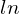cannot take zero as its argument (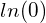is undefined). However, there is quite a high chance that some valuesorbe zero, so in practice, we often add 1 toandwhen computing MSLE. In other words, we often use this modified version of MSLE:

MSLE =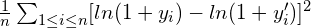Value of MSLE is in range.

For our model to be better, we should minimize MSLE.

MSLE can be used as an Objective function.

Summary

In this blog, we introduced the Objective function and the Evaluation function along with their differences. The Objective function is the target that your model tries to optimize, while the Evaluation function is what we – humans see and care about (and want to optimize).

We also presented some of the most common objective/evaluation functions for regression problems. Each of them has its own advantages and disadvantages.

MAE is simple and easily interpreted.

MSE is an alternative for MAE if you want to emphasize on penalizing higher error.

MaxAE is less common than the 2 above. Use it if you only care about the worst-case scenario.

RMSE is quite similar to MSE. Use it instead of MSE if you want the error to have the same unit as the response.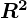, Adjustedand Predictedare used only for linear regression.is the original version, so it is the simplest among the 3. Adjustedpenalizes models that have useless predictors. Predictedis the most robust one against over-fitting.

MSLE should be used when your response is non-negative and is exponential, and you want the error to be proportional to ratio rather than absolute difference. Notice that MSLE penalizes under-estimation more than over-estimation.

So, to close this topic, I would say that choosing which objective/evaluation function to use depends on your specific problem and how you want your outcome to be. You can choose one from above, or just create your own custom function.

References: